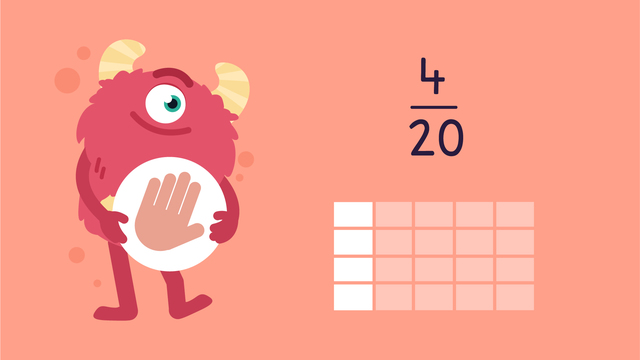# Simplifying Fractions —Let's Practice!Rating

Ø 5.0 / 1 ratings
The authorsTeam Digital
Simplifying Fractions —Let's Practice!
CCSS.MATH.CONTENT.4.NF.A.1

## Basics on the topicSimplifying Fractions —Let's Practice!

Today we are practicing simplifying fractions with Razzi! This video contains examples to help you further practice and grow confident in this area.

### TranscriptSimplifying Fractions —Let's Practice!

Razzi says get these items ready because today we're going to practice Simplifying Fractions It's time to begin! Which fraction is four-twentieths simplified? Two-fifths, one-fifth, one-fourth. Pause the video to work on the problem and press play when you are ready to see the solution! The common factor of four and twenty is four. Four divided by four is one and twenty divided by four is five. Did you also get one-fifth? Let's tackle the next problem! Which fraction is eighteen-twenty-sevenths simplified? Two-thirds,four-fifths, nine-twelfths. Pause the video to work on the problem and press play when you are ready to see the solution! The common factor of eighteen and twenty-seven is nine. Eighteen divided by nine is two and twenty-seven divided by nine is three. Did you also get two-thirds? Let's try one more! What is the simplified fraction of thirty-five, forty seconds? Four-fifths, seven-eighths, five-sixths. Pause the video to work on the problem and press play when you are ready to see the solution! The common factor of thirty-five and forty-two is seven. Thirty-five divided by seven is five and forty-two divided by seven is six. Did you also get five-sixths? Razzi had so much fun practicing with you today! See you next time!

## Simplifying Fractions —Let's Practice! exercise

Would you like to apply the knowledge you’ve learned? You can review and practice it with the tasks for the video Simplifying Fractions —Let's Practice! .
• ### True or False?

Hints

How do we make fractions from the blocks shown in the image?

• Count the number of highlighted blocks in the group on the left. Write this number on the top of the fraction.
• Count the total number of highlighted and non-highlighted blocks on the left. Write this number on the bottom.
Repeat these steps to get the fraction for the blocks on the right.

Equivalent means equal.

To answer question #2, find out if the two fractions in the image are equal.

The greatest common factor is the largest number that both 4 and 12 are divisible by.

Solution

1. True. Left fraction: $\frac{4}{12}$, right fraction: $\frac{1}{3}$
2. True. The two fractions are equivalent.
3. False. The greatest common factor of 4 and 12 is not 2.
• To find the greatest common factor, list the numbers that both 4 and 12 are divisible by. They are: 1, 2 and 4. Therefore, the greatest common factor is 4.

• ### Simplify the fraction.

Hints

List all common factors that the numerator and denominator share. Which is greatest?

Divide the numerator and denominator by the greatest common factor to get the simplified fraction.

Solution

The greatest common factor is 5.

In the simplified fraction, the numerator is 1 and the denominator is 4.

The simplified fraction is $\frac{1}{4}$.

• ### Find the greatest common factor of each fraction.

Hints

List all common factors that the numerator and denominator share.

Choose the largest factor from the list to find the greatest common factor.

The factors of 28 and 35 are:

28: 1, 2, 4, 7, 14

35: 1, 5, 7

What is the largest factor they both share?

Solution

1) $\frac{12}{16}$: The greatest common factor is 4.

2) $\frac{15}{18}$: The greatest common factor is 3.

3) $\frac{28}{35}$: The greatest common factor is 7.

4) $\frac{24}{39}$: The greatest common factor is 3.

• ### Simplify all fractions. Answer the questions.

Hints

Divide the numerator and denominator by the greatest common factor.

List all factors for 35 and 56:

35: 1, 5, 7

56: 1, 2, 4, 7, 8, 14, 28

Divide both numbers by the greatest factor they both share to simplify the fraction.

Solution

$\frac{27}{36}$: The greatest common factor is 9. The simplified fraction is 3 over 4 or $\frac{3}{4}$.

$\frac{20}{28}$: The greatest common factor is 4. The simplified fraction is 5 over 7 or $\frac{5}{7}$.

$\frac{35}{56}$: The greatest common factor is 7. The simplified fraction is 5 over 8 or $\frac{5}{8}$.

• ### Simplify the fraction.

Hints

To find the unsimplified fraction shown by the blocks:

• Count the number of highlighted blocks. Write this number on the top of the fraction.
• Count the total number of highlighted and non-highlighted blocks. Write this number on the bottom.

To find the greatest common factor:

• Count the number of highlighted blocks in the numerator.
• Count the number of highlighted blocks in the denominator.
Which numbers can both the numerator and denominator be divided by?

Divide 4 and 6 by their greatest common factor to find the simplified fraction.

Solution
1. The unsimplified fraction is $\frac{4}{6}$.
2. The greatest common factor is 2.
3. The numerator of the simplified fraction is 2.
4. the denominator of the simplified fraction is 3.
5. The simplified fraction is $\frac{2}{3}$.
• ### Solve the word problem.

Hints

Shown below is a daisy.

Start by writing out the unsimplified fraction.

The number of daisies is the numerator.

The total number of all flowers is the denominator.

Count out the number of daisies and total number of flowers in the photo if you need to.

Next, simplify the fraction.

Divide both the numerator and denominator by the greatest common factor.

Solution

The fraction of daisies in the total bouquet is 3 over 8 or $\frac{3}{8}$.

The unsimplified fraction is $\frac{12}{32}$. Divide each number by 4 to get the simplified fraction, $\frac{3}{8}$.